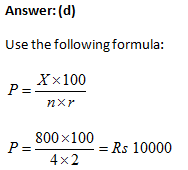Question 1: How much a person should pay per year to payment the debt of Rs 4180 due in 4 years at the rate of 3% per annum?
(a) 1400
(b) 1000
(c) 1300
(d) 1500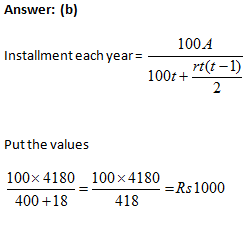Question 2: The sum of interest on a sum of money is 1/36 of the principal, and the number of years is equal to the rate of interest. What will be the rate percent?
(a) 5/3%
(b) 4/3%
(c) 7/3%
(d) 8/3%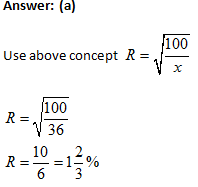Question 3: The rate of interest for 2 years is 3%, 4 years is 6%,2 years is 4%.If a man gets interest of Rs. 3800 for 9 years, Calculate the principal amount.
(a) 14000
(b) 10000
(c) 13000
(d) 15000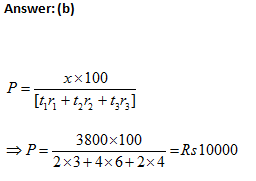Question 4: A sum of money becomes 3 times in 25 years. Calculate the rate of interest.
(a) 8%
(b) 10%
(c) 12%
(d) 13%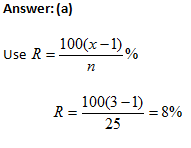Question 5: A sum was put at SI at a certain rate for 4 years. If the sum would have put at a 2% higher rate, it could yield Rs 800 more. What will be the principal?
(a) 14000
(b) 12000
(c) 13000
(d) 10000